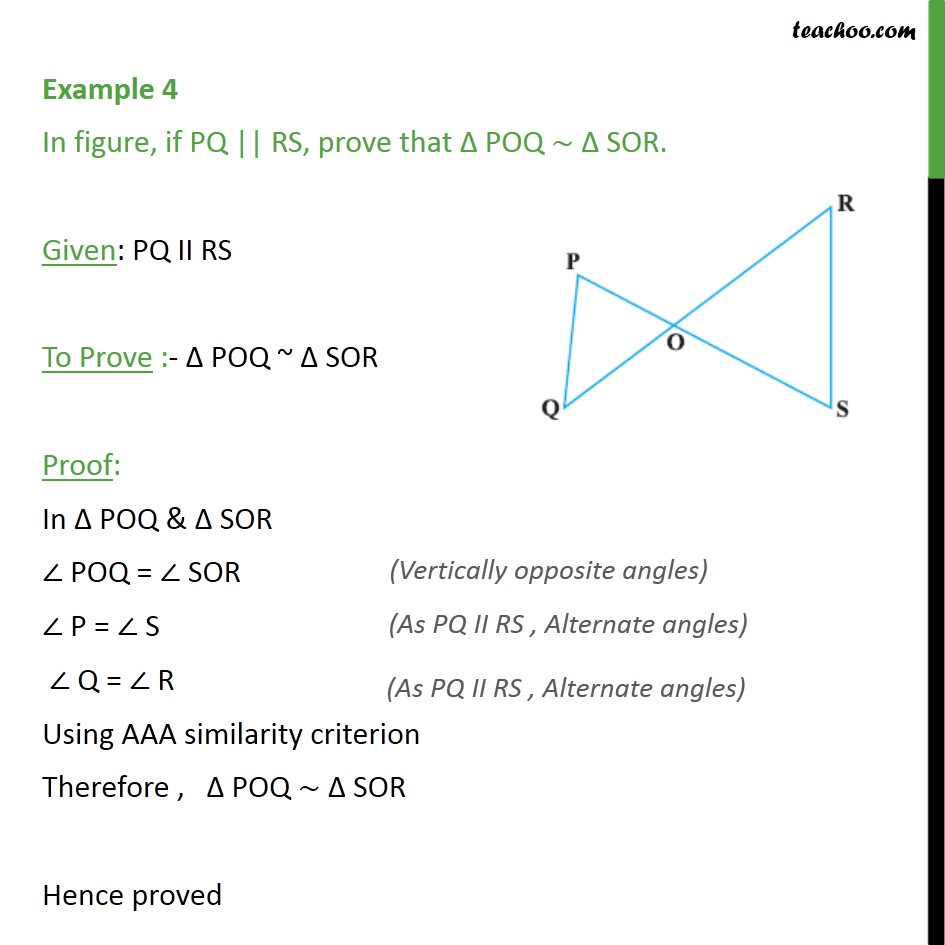Examples

Chapter 6 Class 10 Triangles
Serial order wiseLearn in your speed, with individual attention - Teachoo Maths 1-on-1 Class

### Transcript

Example 4 In figure, if PQ || RS, prove that Δ POQ ~ Δ SOR. Given: PQ II RS To Prove :- Δ POQ ~ Δ SOR Proof: In Δ POQ & Δ SOR ∠ POQ = ∠ SOR (Vertically Opposite Angles) ∠ P = ∠ S (AS PQ || RS, alternate angles) ∠ Q = ∠ R (AS PQ || RS, alternate angles) Using AAA similarity criterion Therefore , Δ POQ ~ Δ SOR Hence proved